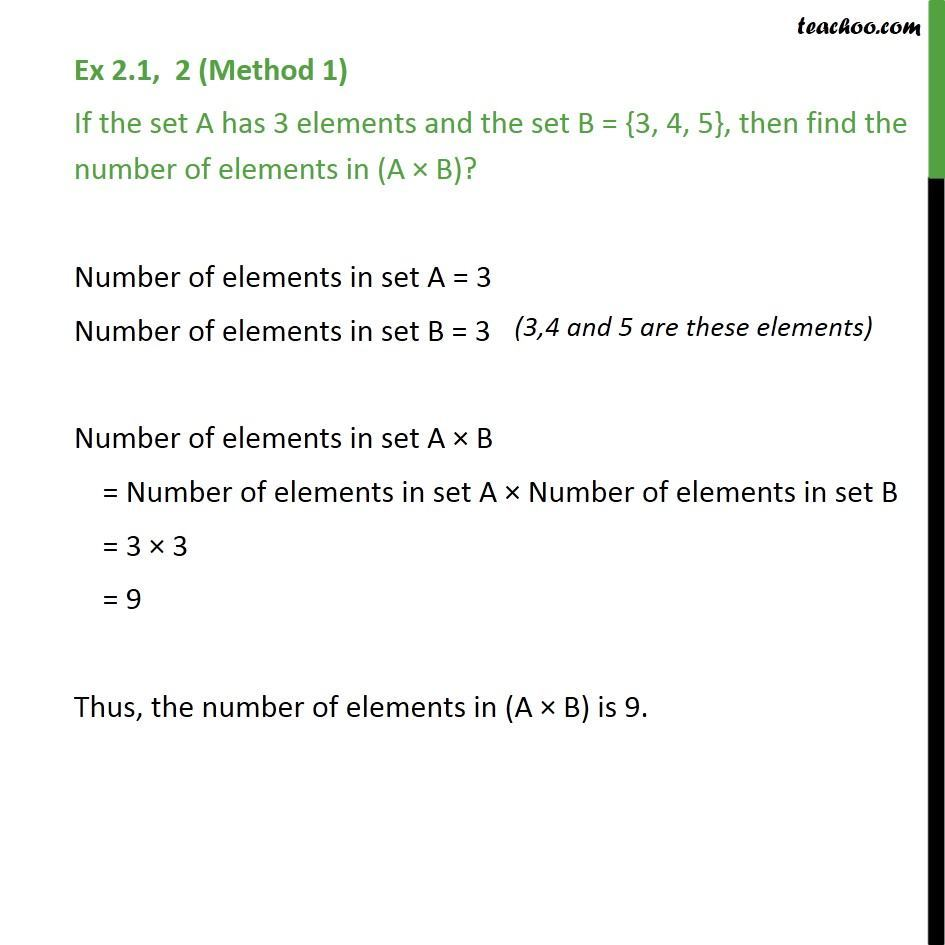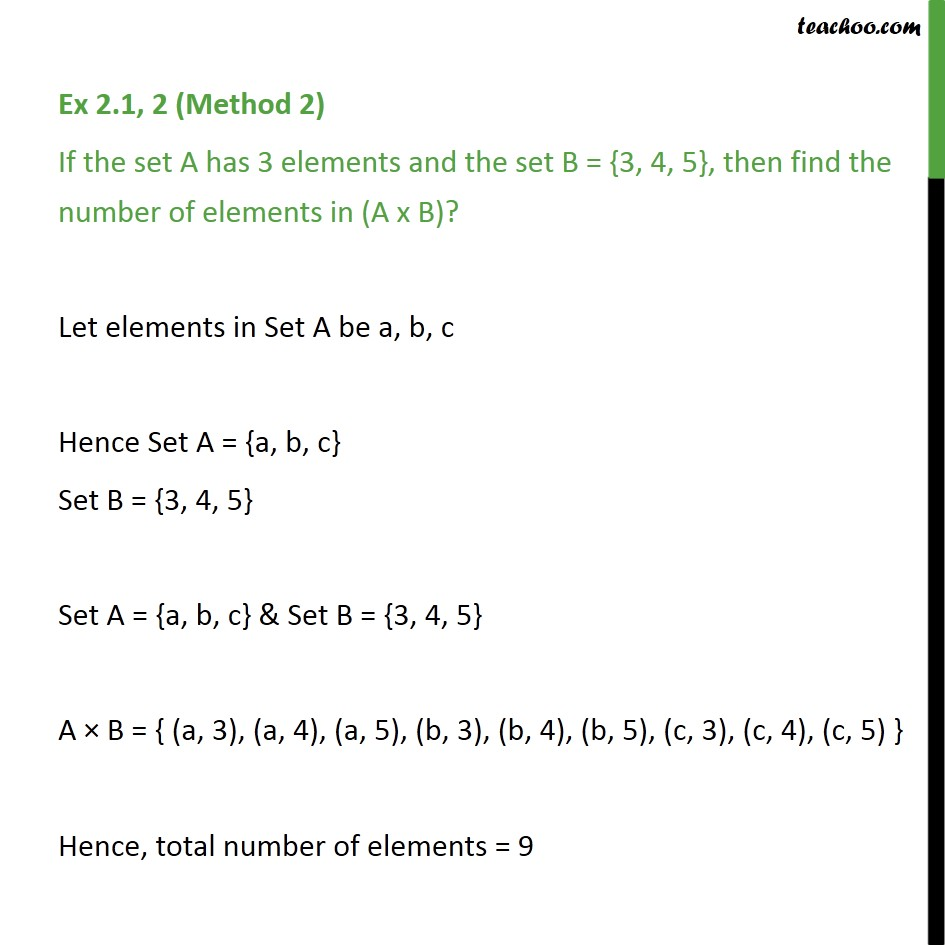1. Chapter 2 Class 11 Relations and Functions
2. Serial order wise
3. Ex 2.1

Transcript

Ex 2.1, 2 (Method 1) If the set A has 3 elements and the set B = {3, 4, 5}, then find the number of elements in (A B)? Number of elements in set A = 3 Number of elements in set B = 3 Number of elements in set A B = Number of elements in set A Number of elements in set B = 3 3 = 9 Thus, the number of elements in (A B) is 9. Ex2.1, 2 (Method 2) If the set A has 3 elements and the set B = {3, 4, 5}, then find the number of elements in (A B)? Let elements in Set A be a, b, c Hence Set A = {a, b, c} Set B = {3, 4, 5} Set A = {a, b, c} & Set B = {3, 4, 5} A B = {(a, 3), (a, 4), (a ,5), (b, 3), (b, 4), (b, 5), (c, 3), (c, 4), (c,5) } Hence, total number of elements = 9

Ex 2.1